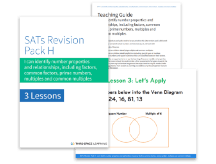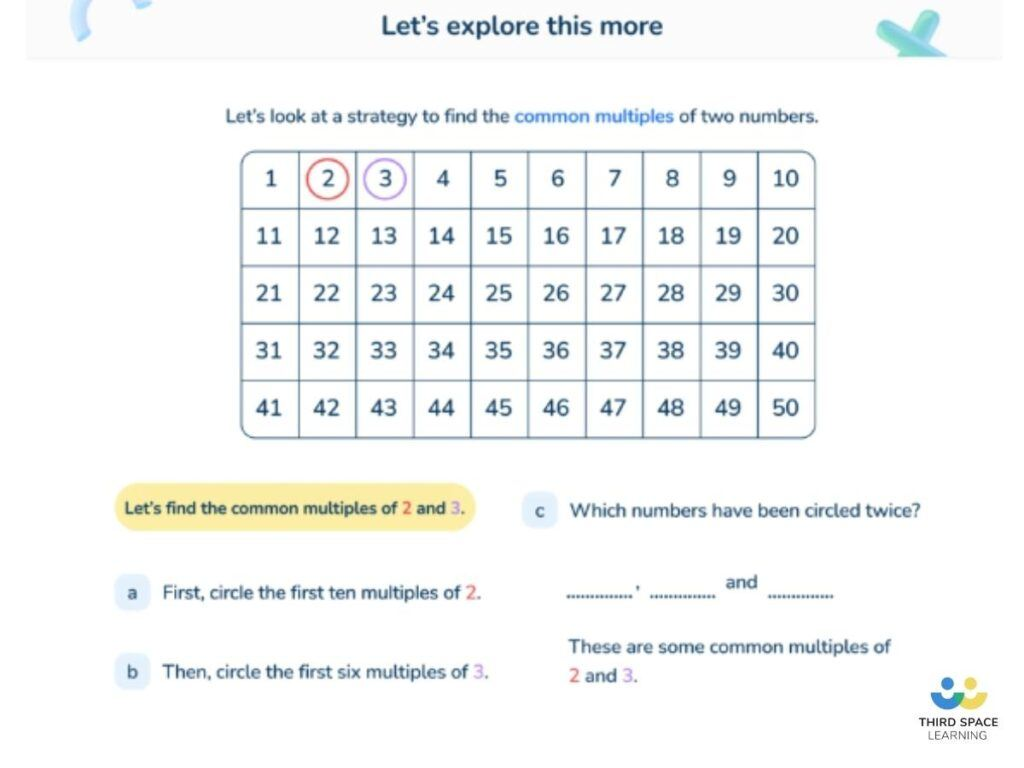Teaching support from the UK’s largest provider of in-school maths tuitionone to one lessonsschools supported

National Tutoring Programme

Heavily subsidised one to one maths lessons designed to plug gaps, build confidence and boost progress

Hundreds of FREE online maths resources!

Daily activities, ready-to-go lesson slides, SATs revision packs, video CPD and more!Explore all resources
Four Operations

National Tutoring Programme

Heavily subsidised one to one maths lessons designed to plug gaps, build confidence and boost progress

# What Is A Multiple: Explained For Primary School

Make learning what is a multiple, simple for KS1 and KS2 children. Here is a step by step guide to support teaching multiples in Primary School. A secure knowledge of times tables is essential in calculating multiples.

### What is a multiple?

A multiple of a number is the result when that number is multiplied by an integer. Examples of multiple of 14 could be 14 (14 x 1), 28 (14 x 2) or 140 (14 x 10). The definition of multiple can also be described as numbers in a given times table – for example, 5, 10, 15 and 20 are all in the 5 times table; they are also all multiples of 5. Even numbers are all multiples of 2. There is a multiple of every number.

Children often confuse multiples with factors. Read more about factors of a number and the greatest common factor (also know as the highest common factor).

### When do children learn about multiples in the national curriculum?

Children are expected to know the first 12 multiples of the numbers 1-12 by the end of Year 4, when they will have their times table check this is gradually taught from the beginning of

Year 1:

• Count in multiples (also known as ‘skip counting’) of twos, fives and tens

Year 2:

• Count in multiples of three to support later understanding of a third

Year 3:

• Count from 0 in multiples of 4, 8, 50 and 100 (pupils now use multiples of 2, 3, 4, 5, 8, 10, 50 and 100)

Year 4:

• Count in multiples of 6, 7, 9, 25 and 1000
• Use factors and multiples to recognise equivalent fractions and simplify where appropriate

Alongside the regular times tables, multiples of 25, 50, 100 and 1000 are also explicitly taught as these are useful when working with fractions decimals percentages and units of measurement

Year 5:

Year 6:

• Identify common factors, common multiples and prime numbers
• Use common multiples to express fractions in the same denomination
• Solve problems involving unequal sharing and grouping using knowledge of fractions and multiplesFactors, Multiples, Square & Cube Number Pack

Download a free pack of SATs revision worksheets with questions on multiples, factors, square and cube numbers.Download Free Now!

### What is the lowest common multiple?

The lowest common multiple (LCM), also referred to as the least common multiple, is the smallest multiple shared between two given numbers. For example, the lowest common multiple of 9 and 12 is 36 as it is the smallest multiple that both given numbers share (9 x 4 = 36 and 12 x 3 = 36). Lowest common multiples can be used to express fractions in the same denomination, which is useful when they are being added or subtracted.A simple visual representation of how to identify common multiples in a Third Space Learning online tutoring lesson.

### How do multiples relate to other areas of maths?

Multiples and factors are often taught together: whilst factors are the result when you divide a number by an integer (with no remainder), multiples are the result when you multiply a number by an integer. In Year 5 geometry, children are taught multiples of 90 degrees (90 = quarter turn; 180 = half a turn, or a straight line; 270 = three-quarter turn; 360 = full turn, or a circle). As previously mentioned, finding common multiples are also useful when expressing fractions in the same denomination.

### Multiples of numbers in real life

Multiples are useful when organising a number into groups – for example, 25 is a multiple of 5: 25 children could be organised into groups of 5; 25p could be paid in 5ps. Common multiples are also useful in real life contexts – for example, if burgers come in packs of 8 but burger buns come in packs of 6, finding a common multiple (24) would help you work out how many of each pack to buy (3 and 4 respectively) without wasting any.

### 5 multiple practice questions and answers

• 1. What’s the lowest common multiple of 8 and 12?
• 2. Chen uses these digit cards: 5 6 9. She makes a two-digit number and a one-digit number. She multiplies them together. Her answer is a multiple of 10. What could Chen’s multiplication be? (SATs 2017)
• 3. Write all the common multiples of 3 and 8 that are less than 50. (SATs 2016)
• 4. In the circles, write a multiple that belongs to each set. One has been done for you. (SATs 2016 sample)
• 5. Here is a diagram for sorting numbers. Write one number in each box. One is done for you. (SATs 2016 sample)

1. 24
2. 96 x 5
3. 24, 48
4. a) 120, 140, 160 or 180
b) 210, 240 or 270
c) 320 or 360
5. Top right: 3, 6, 9, 12, 18 etc.
Bottom left: 5, 10, 20, 25, 35 etc.
Bottom right: 1, 2, 4, 7, 8 etc.

Looking for more multiplication resources? Take a look at our collection of multiplication worksheets.

What are the multiples of 12?

Some of the multiples of 12 are 12, 24, 36, 48, 60, 72, 84, 96, 108, 120, 132, 144, but there are many many more. The multiples of 12 are achieved by multiplying any other number by 12.

What is a multiple and a factor?

A multiple is the product of two numbers. A factor is a number that fits exactly into a given number, or divides a particular number with no remainder. A factor is always a positive integer (whole number).

How do you explain multiples?

A multiple of any given number can be achieved by multiplying that number by any integer (whole number)

Looking for more advice on how to explain other mathematical concepts to your students? Take a look at our Primary Maths Dictionary.

Do you have pupils who need extra support in maths?
Every week Third Space Learning’s maths specialist tutors support thousands of pupils across hundreds of schools with weekly online 1-to-1 lessons and maths interventions designed to plug gaps and boost progress.

Since 2013 we’ve helped over 150,000 primary and secondary school pupils become more confident, able mathematicians. Learn more or request a personalised quote for your school to speak to us about your school’s needs and how we can help.##### Sophie Bartlett
Year 6 Teacher
Sophie teaches mixed age classes at a small school in central England. She is a self confessed grammar pedant and number nerd so we've welcomed her as a regular author and editor for Third Space Learning.

Related Articles

x#### Factors, Multiples, Square & Cube Number Pack

Download a free pack of SATs revision worksheets with questions on multiples, factors, square and cube numbers.

#### Factors, Multiples, Square & Cube Number PackDownload a free pack of SATs revision worksheets with questions on multiples, factors, square and cube numbers.